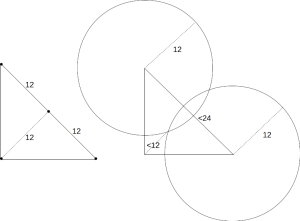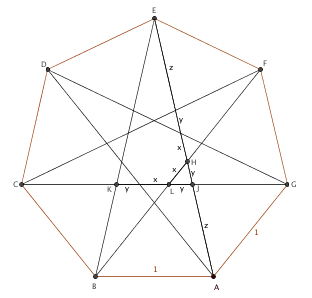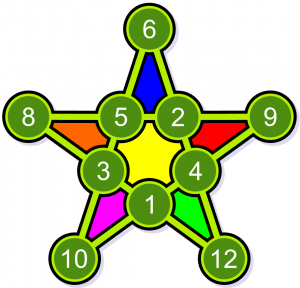##This week, the Varsity Math team is excited by some problems they pondered at halftime during the recent Super Bowl 50. (The relay event is also on break and will return in three weeks.)### Football History

Occasionally you see a football score that allows you to know exactly what happened during the game. For example, if you see that a team is winning 5-0, you know that team scored a field goal and a safety. To simplify things slightly for the sake of this problem, only consider the following possible ways to score: a safety, for two points; a field goal, for three; a touchdown, for six; an extra point, for one, but only immediately after a touchdown; and a 2-point conversion, but only immediately after a touchdown. Only one of an extra point or a 2-point conversion can occur after a given touchdown. Although it’s possible to score two points on defense during a conversion attempt, you should ignore this scenario when solving this problem.

What is the lowest possible score for one team that can be obtained in at least five ways, not counting the order of the scoring plays, but counting safeties as different from 2-point conversions?

[asciimathsf]

### Hail Math-y

Marcus from Varsity Math also plays on the university’s football team as a wide receiver. In one game, he caught a long pass at the center of the other team’s 20-yard line. There was a lone defender in front of him at the center of the 10-yard line. The other defenders were all equally spaced on the 25-yard line on other half of the field (in other words, 55 yards behind Marcus).

The opposing team consisted of math-football geniuses, with perfect strategy. Their actions may depend on what Marcus does. Marcus has one advantage: He runs 12% faster than everyone on the opposing team, all of whom run at equal speed.

All of the players can change direction instantly without losing speed and start running at full speed instantly. Marcus can choose whatever path he likes, but the play is over when he ends up within one yard of a defender (he then gets tackled), he runs out of bounds (the field is 160 feet wide), or he reaches the end zone (the 0-yard line.) His goal, of course, was to run as far toward the end zone as possible.

How deep was Marcus able to get into the opposing team’s territory? Round your answer up to the farthest integer yard line that Marcus passes.

(Thanks to Po-Shen Loh, associate professor of mathematics at Carnegie Mellon, head coach of the United States’ International Math Olympiad team, and founder of Expii for this problem.)

## Solutions to Week 22

Tree-Angle. Whatever the dimension of the park,it is easy to see that the four trees must be planted within the smaller isosceles triangle which is six meters inside the park in all directions, as in the first diagram, so that the circles surrounding their trunks will not extend outside the walls of the park. Therefore, we seek to minimize the size of this inner isosceles triangle, and then we will add 12 + 6√2 to its minimal side length to get the side length needed for the park, as shown in that diagram.

For the inner isosceles triangle, in order for the circles of radius six meters around each trunk not to overlap, it’s equivalent for the trunks to each be at least 12 meters apart from each other, so we will think in terms of that condition. Now note that we may assume without any loss of generality that one of the trees is exactly in one of the acute corners of the inner isosceles triangle: if not, simply take the tree closest to that corner and move it to the corner. That tree can only become farther from its nearest neighbors in such a move, so the conditions will still be satisfied. Similarly, we may assume that another tree is in the other acute corner of the triangle.

Now it is easy to see that all four trees can be placed if the hypotenuse of the isosceles triangle is 24 meters, as in the left illustration of the second diagram below, by placing one tree in each corner of the triangle and one at the midpoint of the hypotenuse. On the other hand, if the hypotenuse is any less than 24 meters long, the circles of radius 12 centered at each of the acute vertices (where we know there are trees) leave a region with diameter less than 12, and so impossible to fit two trees into. Hence, the minimum size of the inner isosceles triangle is for it to have a hypotenuse of 24 meters, and so a leg of at least 24/√2 = 12√2 meters. Adding in the extra from the first paragraph, the shortest park wall must be at least 12+18√2 meters long.Spiral Fleeing. The key to this problem is that the path of the flea (which is nearly impossible to calculate) is a complete red herring. We shall find the distance it travels simply by taking the time it is flying and multiplying by its (constant) speed. Each dog runs one half of the diagonal of the square initially formed by the four dogs. Since the side of the square is 12 + 18√2, the diagonal is 12√2 + 36, and the half-diagonal is 18 + 6√2. At a rate of one meter per second, the dogs take 18 + 6√2 seconds to reach the center and stop. So this is precisely the same length of time that the flea is flying. So at five meters per second, the length of its path is 90 + 30√2 meters.

Heptapiped. The previous answer divided by 30 is 3 + √2, so A is 3 and B is 1.Turning to the heptagram, the diagram shows the heptagon in which it is inscribed, the sides of the heptagram broken into various segments, the middle of which is of length x as described in the problem, and labels some specific points. The plan is to derive enough relations between x, y, and z from the diagram to be able to determine the volume of a rectangular solid with dimensions x, x + 1, and x + 3.

We begin by noting that the angles at the vertices of the heptagram are all π/7, and that by symmetry angles EBC and FBA are equal, and hence both equal to 2π/7 (since the interior angle of a regular heptagon is 5π/7). But then also ∠BAD = 2π/7 and so ∠BAH = 3π/7, and hence ∠BHA = 2π/7. In other words, triangle BHA is isosceles, so z + y = 1. That’s our first relation.

Next, since vertical angles are equal, triangles AJG and HJL are similar. Hence z/1 = y/x, or y = zx. That’s our second relation, and we can also solve z + zx = 1 for z = 1/(1+x).

Finally, since line CG is parallel to AB, triangles BEA and KEJ are similar. That means that (2z+2y+x)/1 = (z+2y+x)/(2y+x). Cross multiplying and substituting for y and using that 2z+2y = 2, we have z+2zx+x = (2+x)(2zx+x). Substituting for z, we have 1/(1+x) + 2x/(1+x) + x = (2+x)(2x/(1+x) + x). Multiplying through by 1+x, this is 1 + 3x + x² = (2+x)(3x+x²). Collecting all of the terms with x on the right hand side, yields 1 = x³ + 4x² + 3x = x(x+1)(x+3). But now the right hand side is precisely the volume we are looking for, so that rectangular solid has volume equal to 1 cubic unit.

Math Star. There are ten spaces to fill in numbers in the pentagram,so the answer must be at least 10; and it is not hard to find a solution with highest number 12, as in the diagram, so the answer is at most 12. So the key to finishing the problem is to determine whether there is a magic pentagram with highest number 10 or 11.

Each number in the magic pentagram appears in two of the lines of four, so the sum of all the lines is twice the sum of all of the numbers in the magic pentagram. Since the lines all have the same sum, that common sum is one fifth of twice the sum of all of the numbers, or two fifths of the sum of all of the numbers. So if there were a magic pentagram with the numbers one through ten, it would have common sum of 22, since the sum of the numbers from one through ten is 55.

There are few enough combinations of four numbers, ten or less, which sum to 22 that we can write them all down:
1+2+9+10  1+3+8+10  1+4+7+10  1+4+8+9  1+5+6+10  1+5+7+9  2+3+7+10  2+3+8+9  2+4+6+10  2+4+7+9  2+5+6+9  2+5+7+8  3+4+6+9  3+4+7+8  3+5+6+8  4+5+6+7
To have a magic pentagram, we would need to select five of these sums (to be the five lines of the pentagram) such that every pair selected shares exactly one number (since every two lines in a pentagram intersect in exactly one point) and every number is used twice. Note that every sum that uses 10 uses either 1 or 2 as well. Hence, to get two copies of 10, we would have to choose one sum with 10 and 1 and one sum with 10 and 2. There are only two of the latter, so suppose we pick 2+3+7+10. Then we must choose 1+5+6+10, because it is the only other sum with 10 that doesn’t share any other number. But then to get two copies of 1, we would have to choose 1+4+8+9, because it is the only other sum with 1 which does not share two numbers with either of the prior choices. And now we are stuck, because every other sum that includes 3 shares at least two numbers with one of 2+3+7+10, 1+5+6+10, or 1+4+8+9. We get stuck in the same way if we choose 2+4+6+10 instead, because then 1+3+8+10 is forced, then 1+5+7+9, and now there is no second line for 3 available.

Hence, there is no magic pentagram with largest number 10. What about 11? In this case, the sum of the numbers can range from 56 (if we leave out the 10) to 65 (if we leave out the 1). Since the common sum is two fifths of the sum of all of the numbers, the magic pentagram must either leave out 6 and have a common sum of 24 or leave out 1 and have a common sum of 26. But the latter case is impossible, because if it did work, we could subtract 1 from every entry and get a magic pentagram using the numbers 1 through 10 with a common sum of 22, which we have already shown impossible.

So the last possibility to check is a magic pentagram using 1,2,3,4,5,7,8,9,10,11 with a common sum of 24. But just as with one to ten summing to 22, there are 16 possible lines, produced simply by substituting 6 by 7, 7 by 8, 8 by 9, 9 by 10, and 10 by 11 in the list above, since every one of the sixteen sums listed has exactly two numbers in it greater than five. Thus the exact same obstruction as observed in that case will occur, simply by adding one to every number bigger than 5 that occurs in the description of that obstruction.

Therefore, a magic pentagram with whole number entries must contain at least a 12 in it somewhere.

## Recent Weeks

Links to all of the puzzles and solutions are on the Complete Varsity Math page.

Come back next week for answers and more puzzles.